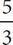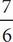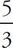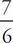# SAT Math Multiple Choice Question 96: Answer and Explanation

### Test Information

Question: 96

6. -24 - 8j = 12k
3 +k = -j

Which of the following ordered pairs (j, k) is the solution to the system of equations above?

• A. (6, -6)
• B. (3, 0)
• C. (0, 2)
• D. (-4, 1)

A Whenever there are variables in the question and numbers in the answer choices, think Plugging In the Answers. In (A), j = 6, and k = -6. Plug these two values into the first equation to get -24 - 8(6) = 12(-6). Solve for both sides of the equation to get -24 - 48 = -72, or -72 = -72. Therefore, the values work for the first equation. Plug the values into the second equation to get 3 +(-6) = -(6). Solve both sides of the equation to get 3 + (-10) = -7, or -7 = -7. Since the values given in (A) work in both equations, the correct answer is (A).